# Sqc-charts

Mar. 18, 2012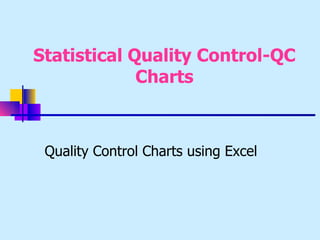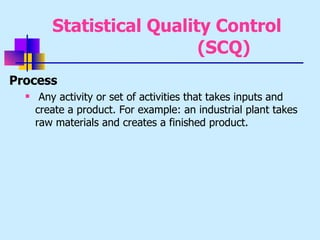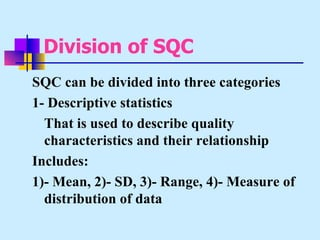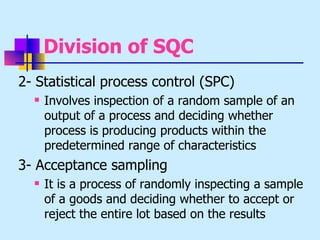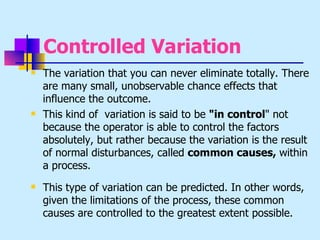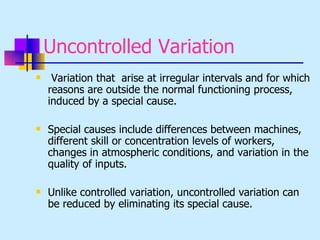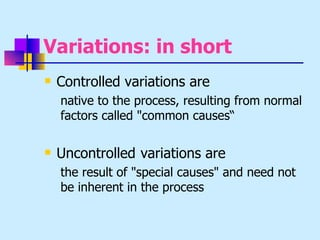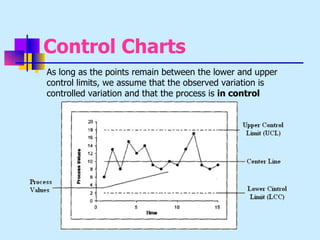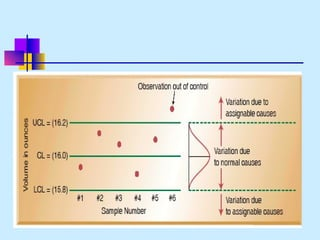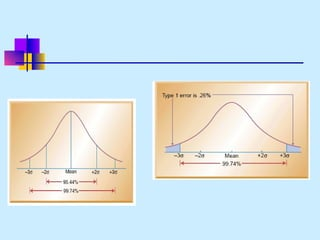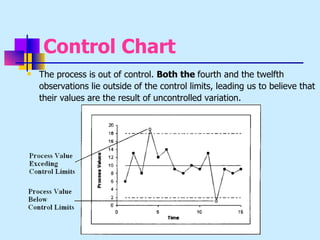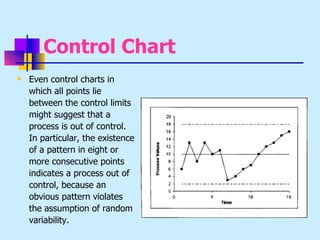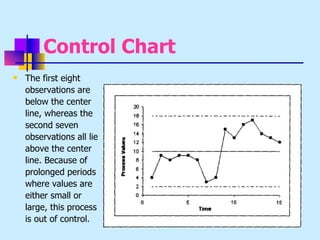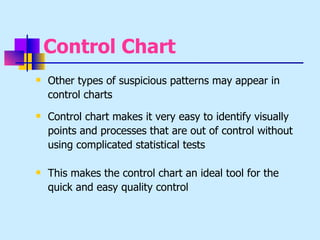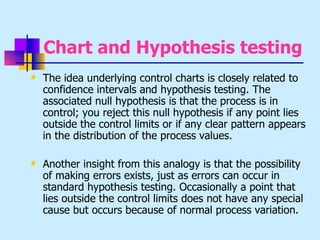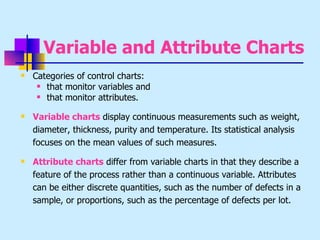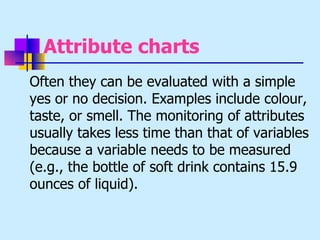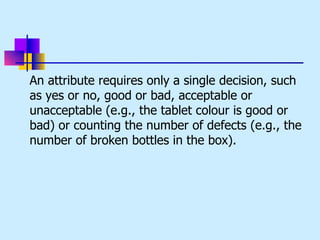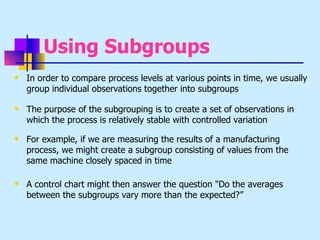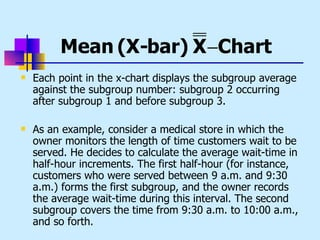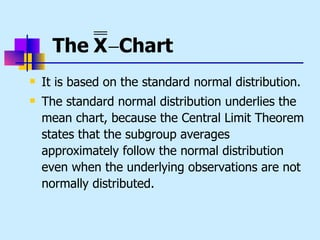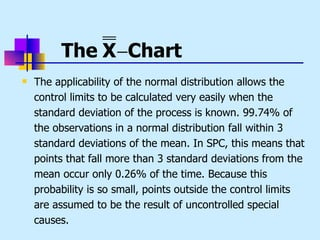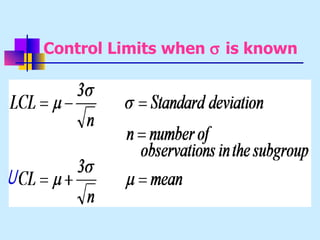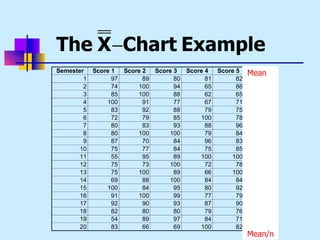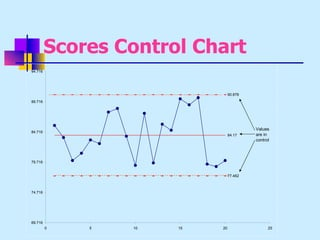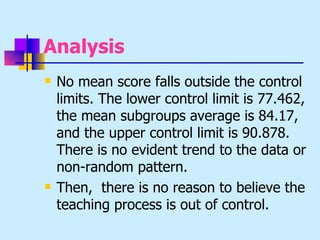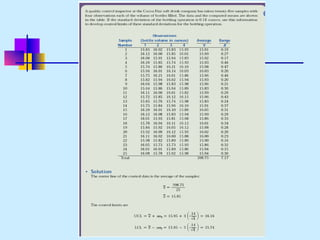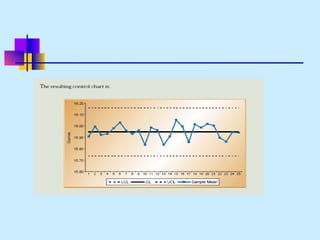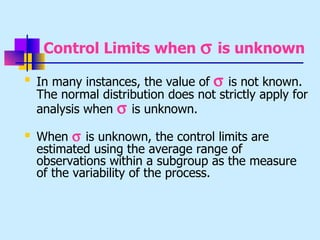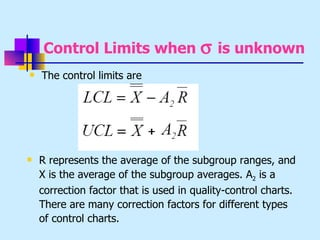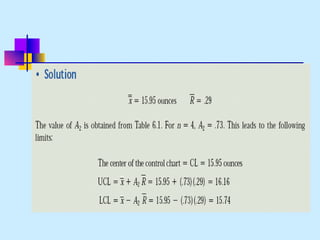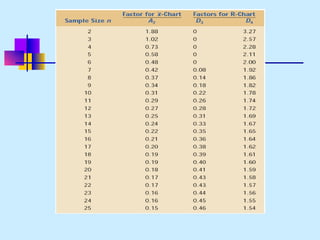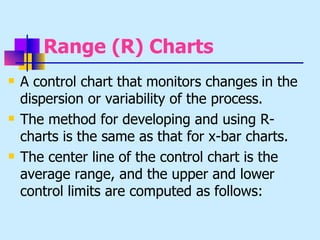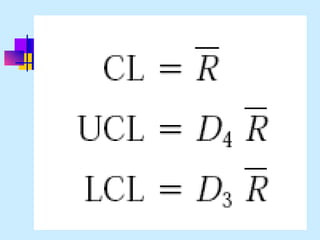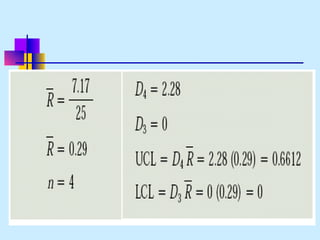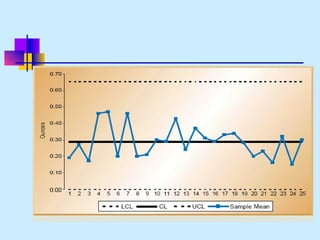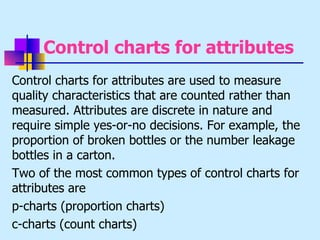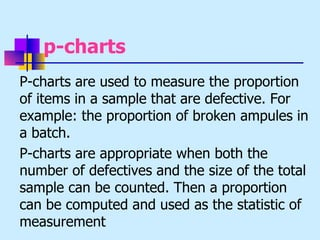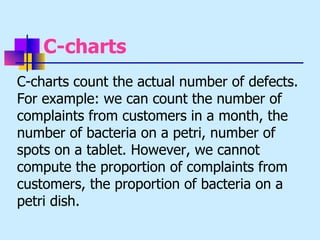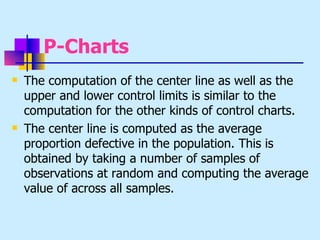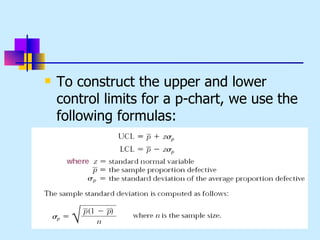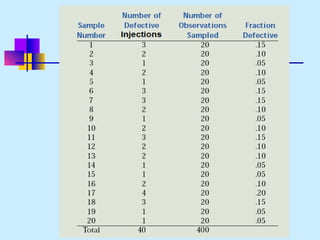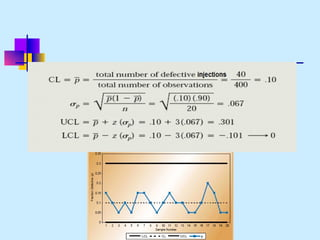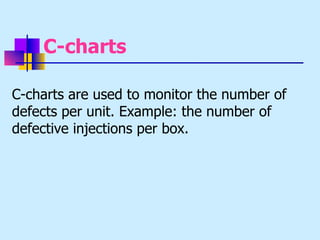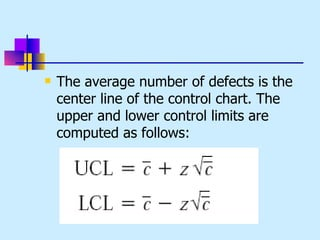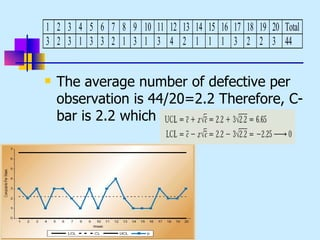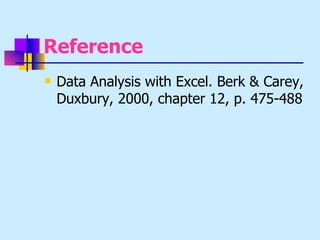1 of 47

### Sqc-charts

1. Statistical Quality Control-QC Charts Quality Control Charts using Excel
2. Statistical Quality Control (SCQ) Process  Any activity or set of activities that takes inputs and create a product. For example: an industrial plant takes raw materials and creates a finished product.
3. Division of SQC SQC can be divided into three categories 1- Descriptive statistics That is used to describe quality characteristics and their relationship Includes: 1)- Mean, 2)- SD, 3)- Range, 4)- Measure of distribution of data
4. Division of SQC 2- Statistical process control (SPC)  Involves inspection of a random sample of an output of a process and deciding whether process is producing products within the predetermined range of characteristics 3- Acceptance sampling  It is a process of randomly inspecting a sample of a goods and deciding whether to accept or reject the entire lot based on the results
5. Controlled Variation  The variation that you can never eliminate totally. There are many small, unobservable chance effects that influence the outcome.  This kind of variation is said to be "in control" not because the operator is able to control the factors absolutely, but rather because the variation is the result of normal disturbances, called common causes, within a process.  This type of variation can be predicted. In other words, given the limitations of the process, these common causes are controlled to the greatest extent possible.
6. Uncontrolled Variation  Variation that arise at irregular intervals and for which reasons are outside the normal functioning process, induced by a special cause.  Special causes include differences between machines, different skill or concentration levels of workers, changes in atmospheric conditions, and variation in the quality of inputs.  Unlike controlled variation, uncontrolled variation can be reduced by eliminating its special cause.
7. Variations: in short  Controlled variations are native to the process, resulting from normal factors called "common causes“  Uncontrolled variations are the result of "special causes" and need not be inherent in the process
8. Control Charts  As long as the points remain between the lower and upper control limits, we assume that the observed variation is controlled variation and that the process is in control
9. Control Chart  The process is out of control. Both the fourth and the twelfth observations lie outside of the control limits, leading us to believe that their values are the result of uncontrolled variation.
10. Control Chart  Even control charts in which all points lie between the control limits might suggest that a process is out of control. In particular, the existence of a pattern in eight or more consecutive points indicates a process out of control, because an obvious pattern violates the assumption of random variability.
11. Control Chart  The first eight observations are below the center line, whereas the second seven observations all lie above the center line. Because of prolonged periods where values are either small or large, this process is out of control.
12. Control Chart  Other types of suspicious patterns may appear in control charts  Control chart makes it very easy to identify visually points and processes that are out of control without using complicated statistical tests  This makes the control chart an ideal tool for the quick and easy quality control
13. Chart and Hypothesis testing  The idea underlying control charts is closely related to confidence intervals and hypothesis testing. The associated null hypothesis is that the process is in control; you reject this null hypothesis if any point lies outside the control limits or if any clear pattern appears in the distribution of the process values.  Another insight from this analogy is that the possibility of making errors exists, just as errors can occur in standard hypothesis testing. Occasionally a point that lies outside the control limits does not have any special cause but occurs because of normal process variation.
14. Variable and Attribute Charts  Categories of control charts:  that monitor variables and  that monitor attributes.  Variable charts display continuous measurements such as weight, diameter, thickness, purity and temperature. Its statistical analysis focuses on the mean values of such measures.  Attribute charts differ from variable charts in that they describe a feature of the process rather than a continuous variable. Attributes can be either discrete quantities, such as the number of defects in a sample, or proportions, such as the percentage of defects per lot.
15. Attribute charts Often they can be evaluated with a simple yes or no decision. Examples include colour, taste, or smell. The monitoring of attributes usually takes less time than that of variables because a variable needs to be measured (e.g., the bottle of soft drink contains 15.9 ounces of liquid).
16. An attribute requires only a single decision, such as yes or no, good or bad, acceptable or unacceptable (e.g., the tablet colour is good or bad) or counting the number of defects (e.g., the number of broken bottles in the box).
17. Using Subgroups  In order to compare process levels at various points in time, we usually group individual observations together into subgroups  The purpose of the subgrouping is to create a set of observations in which the process is relatively stable with controlled variation  For example, if we are measuring the results of a manufacturing process, we might create a subgroup consisting of values from the same machine closely spaced in time  A control chart might then answer the question "Do the averages between the subgroups vary more than the expected?”
18. Mean (X-bar) X −Chart  Each point in the x-chart displays the subgroup average against the subgroup number: subgroup 2 occurring after subgroup 1 and before subgroup 3.  As an example, consider a medical store in which the owner monitors the length of time customers wait to be served. He decides to calculate the average wait-time in half-hour increments. The first half-hour (for instance, customers who were served between 9 a.m. and 9:30 a.m.) forms the first subgroup, and the owner records the average wait-time during this interval. The second subgroup covers the time from 9:30 a.m. to 10:00 a.m., and so forth.
19. The X −Chart  It is based on the standard normal distribution.  The standard normal distribution underlies the mean chart, because the Central Limit Theorem states that the subgroup averages approximately follow the normal distribution even when the underlying observations are not normally distributed.
20. The X −Chart  The applicability of the normal distribution allows the control limits to be calculated very easily when the standard deviation of the process is known. 99.74% of the observations in a normal distribution fall within 3 standard deviations of the mean. In SPC, this means that points that fall more than 3 standard deviations from the mean occur only 0.26% of the time. Because this probability is so small, points outside the control limits are assumed to be the result of uncontrolled special causes.
21. Control Limits when σ is known
22. The X −Chart Example Semester Score 1 Score 2 Score 3 Score 4 Score 5 Mean 1 97 89 80 81 82 2 74 100 94 65 86 3 85 100 88 62 65 4 100 91 77 67 71 5 83 92 88 79 75 6 72 79 85 100 78 7 80 83 93 88 96 8 80 100 100 79 84 9 87 70 84 96 83 10 75 77 84 75 85 11 55 95 89 100 100 12 75 73 100 72 78 13 75 100 89 66 100 14 69 88 100 84 84 15 100 84 95 80 92 16 91 100 99 77 79 17 92 90 93 87 90 18 82 80 80 79 76 19 54 89 97 84 71 20 83 66 69 100 82 Mean/n
23. Scores Control Chart 94.716 90.878 89.716 Values 84.716 84.17 are in control 79.716 77.462 74.716 69.716 0 5 10 15 20 25
24. Analysis  No mean score falls outside the control limits. The lower control limit is 77.462, the mean subgroups average is 84.17, and the upper control limit is 90.878. There is no evident trend to the data or non-random pattern.  Then, there is no reason to believe the teaching process is out of control.
25. Control Limits when σ is unknown  In many instances, the value of σ is not known. The normal distribution does not strictly apply for analysis when σ is unknown.  When σ is unknown, the control limits are estimated using the average range of observations within a subgroup as the measure of the variability of the process.
26. Control Limits when σ is unknown  The control limits are  R represents the average of the subgroup ranges, and X is the average of the subgroup averages. A2 is a correction factor that is used in quality-control charts. There are many correction factors for different types of control charts.
27. Range (R) Charts  A control chart that monitors changes in the dispersion or variability of the process.  The method for developing and using R- charts is the same as that for x-bar charts.  The center line of the control chart is the average range, and the upper and lower control limits are computed as follows:
28. Control charts for attributes Control charts for attributes are used to measure quality characteristics that are counted rather than measured. Attributes are discrete in nature and require simple yes-or-no decisions. For example, the proportion of broken bottles or the number leakage bottles in a carton. Two of the most common types of control charts for attributes are p-charts (proportion charts) c-charts (count charts)
29. p-charts P-charts are used to measure the proportion of items in a sample that are defective. For example: the proportion of broken ampules in a batch. P-charts are appropriate when both the number of defectives and the size of the total sample can be counted. Then a proportion can be computed and used as the statistic of measurement
30. C-charts C-charts count the actual number of defects. For example: we can count the number of complaints from customers in a month, the number of bacteria on a petri, number of spots on a tablet. However, we cannot compute the proportion of complaints from customers, the proportion of bacteria on a petri dish.
31. P-Charts  The computation of the center line as well as the upper and lower control limits is similar to the computation for the other kinds of control charts.  The center line is computed as the average proportion defective in the population. This is obtained by taking a number of samples of observations at random and computing the average value of across all samples.
32. To construct the upper and lower control limits for a p-chart, we use the following formulas:
33. C-charts C-charts are used to monitor the number of defects per unit. Example: the number of defective injections per box.
34. The average number of defects is the center line of the control chart. The upper and lower control limits are computed as follows:
35. 1 2 3 4 5 6 7 8 9 10 11 12 13 14 15 16 17 18 19 20 Total 3 2 3 1 3 3 2 1 3 1 3 4 2 1 1 1 3 2 2 3 44  The average number of defective per observation is 44/20=2.2 Therefore, C- bar is 2.2 which is CL
36. Reference  Data Analysis with Excel. Berk & Carey, Duxbury, 2000, chapter 12, p. 475-488